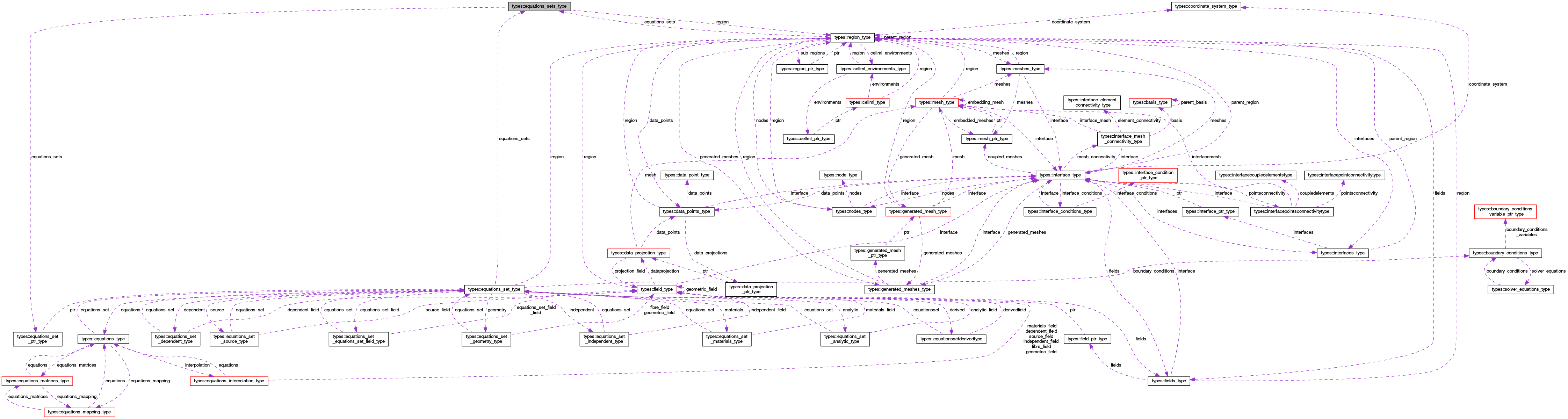OpenCMISS-Iron Internal API Documentation
types::equations_sets_type Type Reference
Collaboration diagram for types::equations_sets_type:[legend]

## Public Attributes

type(region_type), pointer region
A pointer to the region containing the equations sets. More...

integer(intg) number_of_equations_sets
The number of equations sets. More...

type(equations_set_ptr_type), dimension(:), pointer equations_sets
EQUATIONS_SETS(equations_set_idx). EQUATIONS_SETS(equations_set_idx)PTR is the pointer to the equations_set_idx'th equations set. More...

## Detailed Description

Definition at line 1966 of file types.f90.

## Member Data Documentation

 type(equations_set_ptr_type), dimension(:), pointer types::equations_sets_type::equations_sets

EQUATIONS_SETS(equations_set_idx). EQUATIONS_SETS(equations_set_idx)PTR is the pointer to the equations_set_idx'th equations set.

Definition at line 1969 of file types.f90.

 integer(intg) types::equations_sets_type::number_of_equations_sets

The number of equations sets.

Definition at line 1968 of file types.f90.

 type(region_type), pointer types::equations_sets_type::region

A pointer to the region containing the equations sets.

Definition at line 1967 of file types.f90.